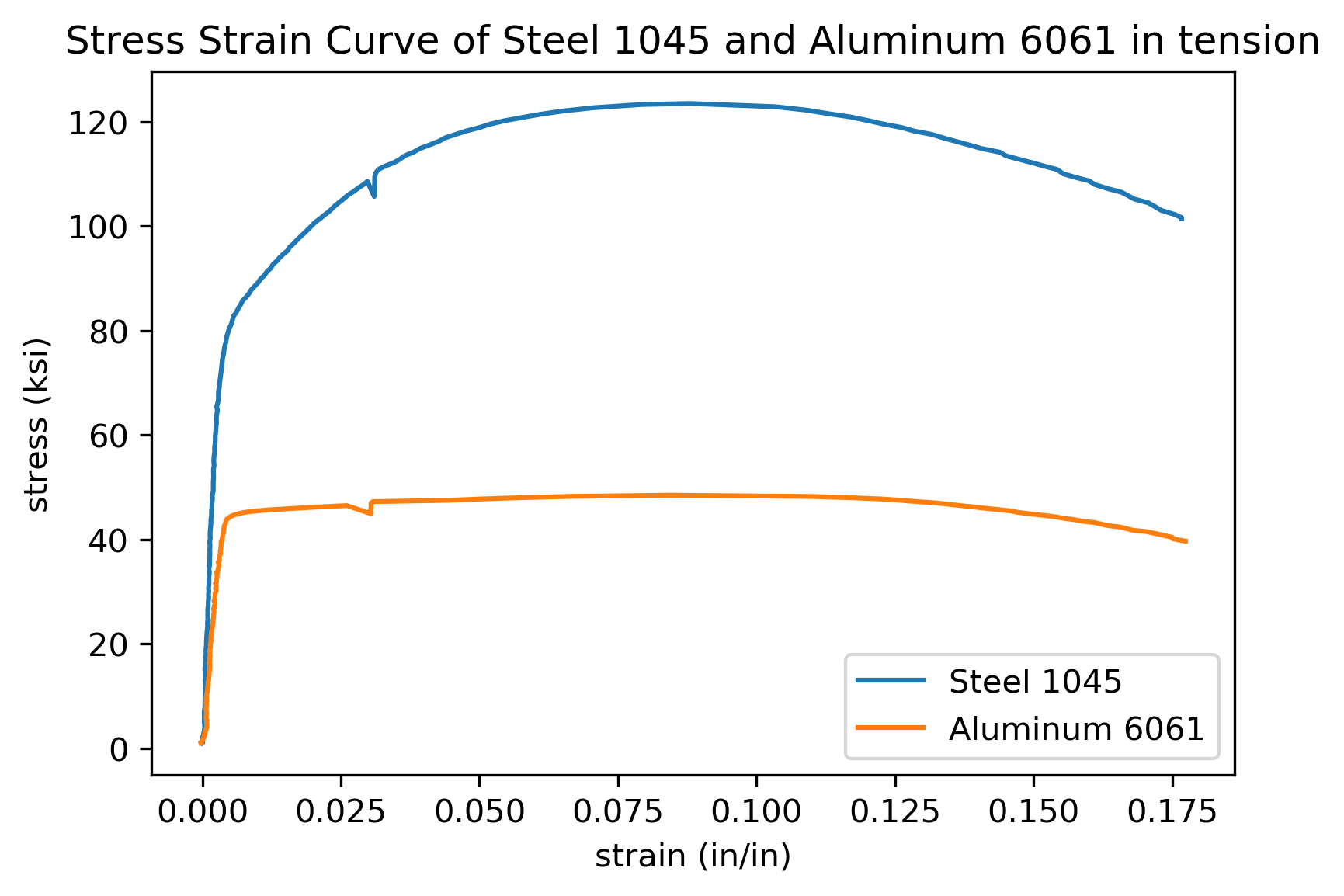## Tensile Test

What key mechanical properties can be obtained from a tensile test curve?

HintHint 2
There are four key mechanical properties obtained from a tensile test curve.
There are four key mechanical properties obtained from a tensile test curve:
• Elastic modulus
• Ductility (also called percent elongation), which is the permanent engineering strain after failure
• Ultimate tensile strength (also called tensile strength), which is the maximum engineering stress
• Yield strength, which is the engineering stress at which permanent deformation is first observed, calculated by 0.2% offset method.
Elastic modulus, Ductility, Ultimate tensile strength, and Yield strength